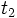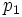# Newton's second law of motion

## Statement

### In terms of force and acceleration

The net external force on a system is the product of the total mass of the system and the acceleration of its center of mass (here, mass is a scalar quantity and both force and acceleration are vector quantities). In symbols:$\overline{F} = m\overline{a}$.

In particular, the direction of the net external force is the same as the direction of acceleration of the center of mass.

For a point mass or particle, we can talk of the acceleration of the whole body, and thus$\overline{a}$ in the above refers to the acceleration of the point mass.

### In terms of momentum and impulse

The net external impulse exerted on a system over a time period equals the difference between the final and initial values of its linear momentum. In other words, if the impulse from time$t_1$ to$t_2$ is$I$, the momentum at time$t_1$ is$p_1$, and the momentum at time$t_2$ is$p_2$, then:$I = p_2 - p_1$.